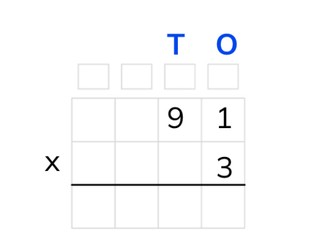Standard algorithm multiplication with a number to 100

# Standard algorithm multiplication with a number to 100

Students learn standard algorithm multiplication with a number to 100.

No account needed.8,000 schools use Gynzy92,000 teachers use Gynzy1,600,000 students use Gynzy

## General

The students learn standard algorithm multiplication with a number to 100.

## Standards

CCSS.Math.Content.5.NBT.B.5

## Learning objective

Students will be able to multiply using the standard algorithm with a number less than 10 and a number to 100.

## Introduction

The students solve the multiplication problems. First with ones, then with tens numbers. In the other exercise they take the multiplication problem out of the story and then solve it.

## Instruction

Explain that with multiplication you can put the numbers one under the other and multiply them working from right to left. This is called standard algorithm multiplication. Show that above each column there is a box for regrouping. In these boxes you can write down the numbers that are carried over and add them to the other outcomes later. Show the example of how you set up the problem 91 x 3. You start multiplying using the standard algorithm. First you multiply the numbers in the ones column together. 3 x 1 = 3. After that you multiply with the number in the tens column. The problem then is 3 x 90 = 270. Now you add up the answers in the intermediate step. 270 + 3 = 273. You write this answer under the line. Emphasize that you don't write down the intermediate step, but fill in the numbers directly under the line. Now solve the two problems together with the students. You may let the students use scrap paper to write down the intermediate steps. Next the students solve the following three problems on their own.

Check whether the students can multiply using the standard algorithm with a number to 100, by asking the following question:
- What steps do you take to solve the problem 32 × 4 using the standard algorithm?

## Quiz

The students test their understanding of standard algorithm multiplication with a number to 100 through ten exercises. For some of these the numbers are already set up in the chart, and for others the students must fill in the chart and solve the problem.

## Closing

Discuss once again the importance of being able to multiply using the standard algorithm. As a closing activity the students solve a few more problems using the standard algorithm. Next you can have the students work together in pairs to find the numbers under the ink spots and complete the problem.

## Teaching tips

Have students that have difficulty with standard algorithm multiplication first practice writing the numbers one under the other. Show how you first make a multiplication problem with the numbers in the ones column and then in the tens column. Emphasize that you shouldn't forget that you have to put a 0 behind the number in the tens column. Next show how you can also directly put the number under the line. Start by practicing this with multiplication problems from the 2 times table.

### The online teaching platform for interactive whiteboards and displays in schools

• Save time building lessons

• Manage the classroom more efficiently

• Increase student engagement